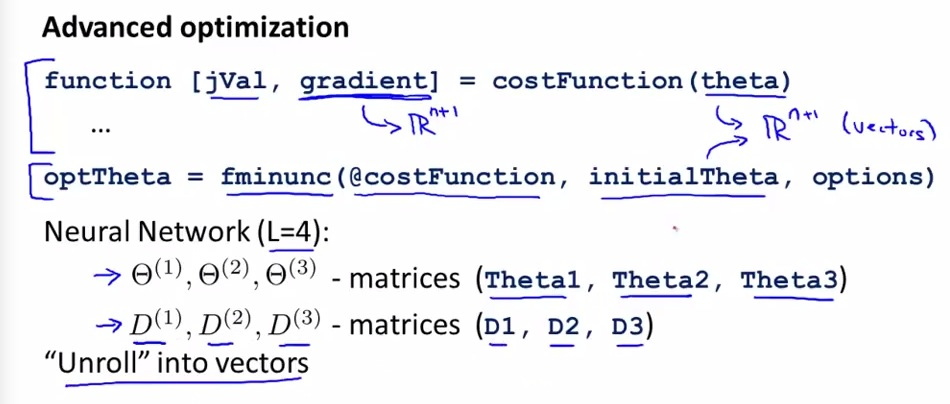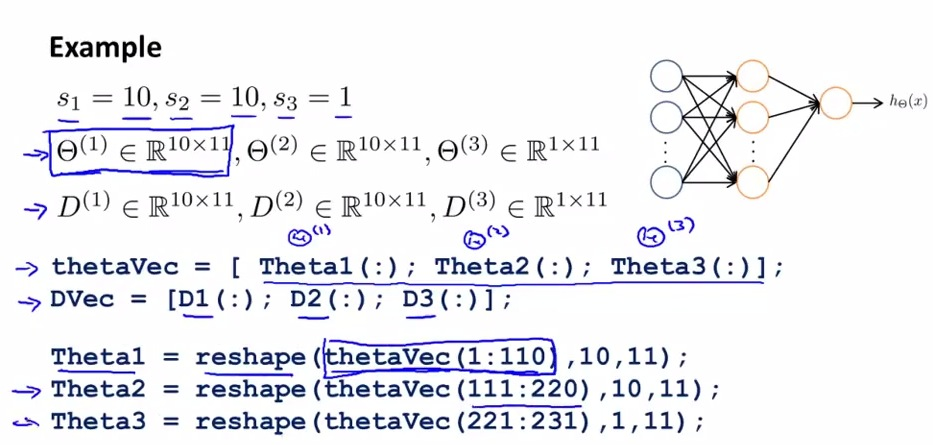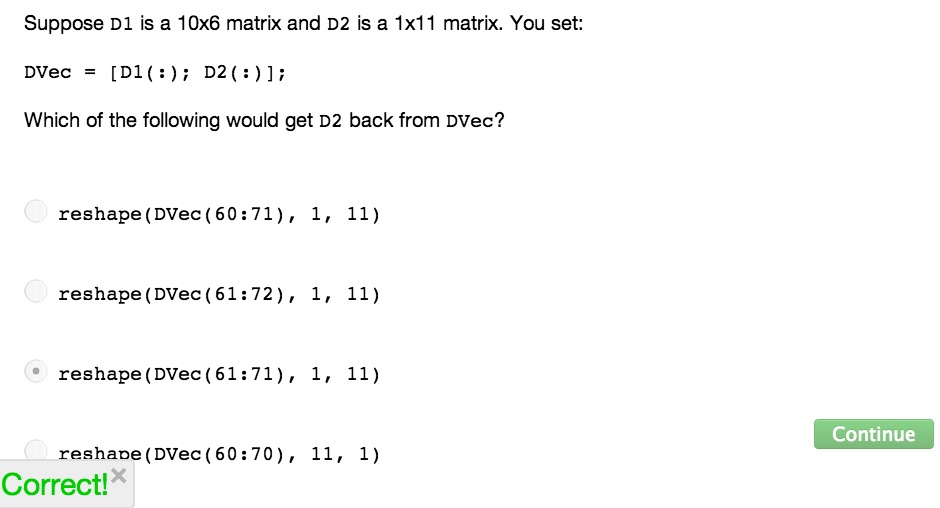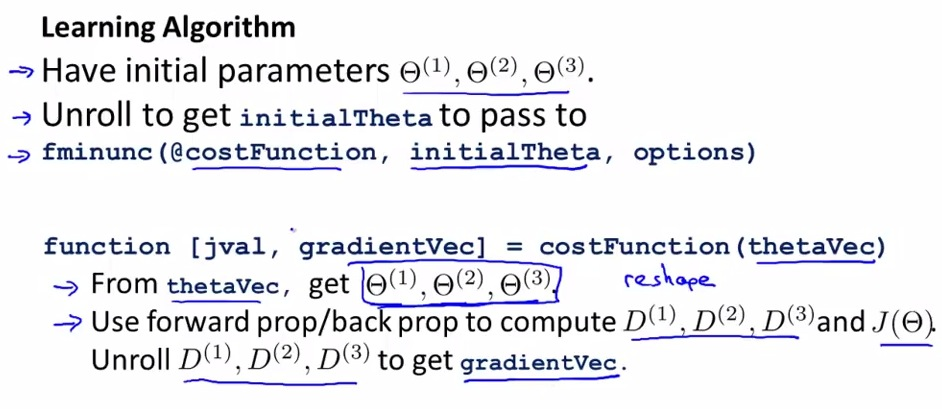• Advanced optimization to unroll parameter from matrices into vectorstheta becomes vector, not a single unit

• unroll so advanced optimization can receive vectors of theta as an input• Reshape: takes number of elemen from a vector, 1:110 (Because theta1 = 10x11) and reshape as one matrices with 10x11 shape• so that's it for matrix vs vector representation
• Advantage matrices: more convenient forward back prop for vectorized implementation
• Advantage vector = vectorized assume dvec,thetavec as just one big vector